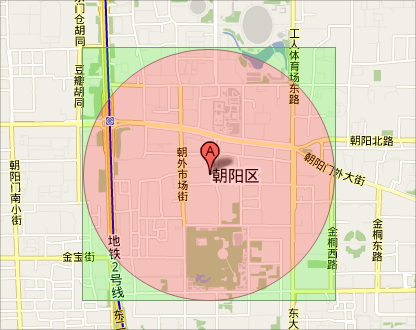MYSQL本身是支持空间索引的，但是在5.x的版本中，取消了对Distance()和Related()的支持，参考这里：MySQL 5.1参考手册 :: 19. 中的空间扩展 19.5.6. 测试几何类之间空间关系的函数，无法使用空间的距离函数去直接去查询距离在一定范围内的点。所以，我首先想到的是，对每条记录，去进行遍历，跟数据库中的每一个点进行距离计算，当距离小于500米时，认为匹配。这样做确实能够得到结果，但是效率极其低下，因为每条记录都要去循环匹配40W条数据，其消耗的时间可想而知。经过记录，发现每条记录处理的时间消耗达到1700ms，针对每天上亿的数据量，这样一个处理速度，让人情何以堪啊。。。红色部分为要求的搜索范围，绿色部分我们能间接得到的结果范围```//\$lat 已知点的纬度
\$dlng =  2 * asin(sin(\$distance / (2 * EARTH_RADIUS)) / cos(deg2rad(\$lat)));
\$dlng = rad2deg(\$dlng);//转换弧度``````\$dlat = \$distance/EARTH_RADIUS;//EARTH_RADIUS地球半径
\$dlat = rad2deg(\$dlat);//转换弧度```

left-top : (lat + dlat, lng – dlng)
right-top : (lat + dlat, lng + dlng)
left-bottom : (lat – dlat, lng – dlng)
right-bottom: (lat – dlat, lng + dlng)

```/**
*计算某个经纬度的周围某段距离的正方形的四个点
*
*@param lng float 经度
*@param lat float 纬度
*@param distance float 该点所在圆的半径，该圆与此正方形内切，默认值为10千米
*@return array 正方形的四个点的经纬度坐标
*/
function returnSquarePoint(\$lng, \$lat,\$distance = 10)
{
\$radius = 6371; //地球半径，平均半径为6371km

\$dlng =  2 * asin(sin(\$distance / (2 * \$radius)) / cos(deg2rad(\$lat)));
\$dlng = rad2deg(\$dlng);

\$dlat = \$distance/\$radius;
\$dlat = rad2deg(\$dlat);

return array(
'left-top'=>array('lat'=>\$lat + \$dlat,'lng'=>\$lng-\$dlng),
'right-top'=>array('lat'=>\$lat + \$dlat, 'lng'=>\$lng + \$dlng),
'left-bottom'=>array('lat'=>\$lat - \$dlat, 'lng'=>\$lng - \$dlng),
'right-bottom'=>array('lat'=>\$lat - \$dlat, 'lng'=>\$lng + \$dlng)
);
}

\$squares = returnSquarePoint(\$lng, \$lat);
\$sql = select *,((ACOS(SIN(\$lat * PI() / 180) * SIN(`lat_baidu` * PI() / 180) + COS(\$lat * PI() / 180) * COS(`lat_baidu` * PI() / 180) * COS((\$lng-`lng_baidu`) * PI() / 180)) * 180 / PI()) * 111.18957696) AS distance from `sales_service_point` where lat_baidu<>0 and lat_baidu>{\$squares['right-bottom']['lat']} and lat_baidu<{\$squares['left-top']['lat']} and lng_baidu>{\$squares['left-top']['lng']} and lng_baidu<{\$squares['right-bottom']['lng']} and status = 1 order by distance asc limit 10```